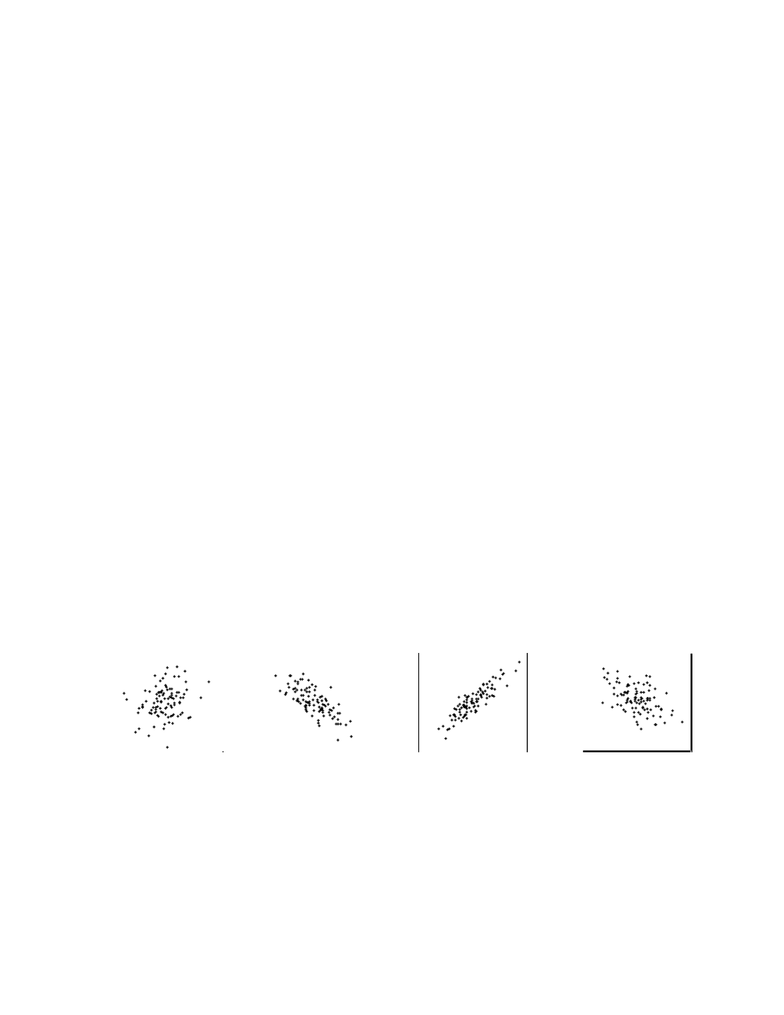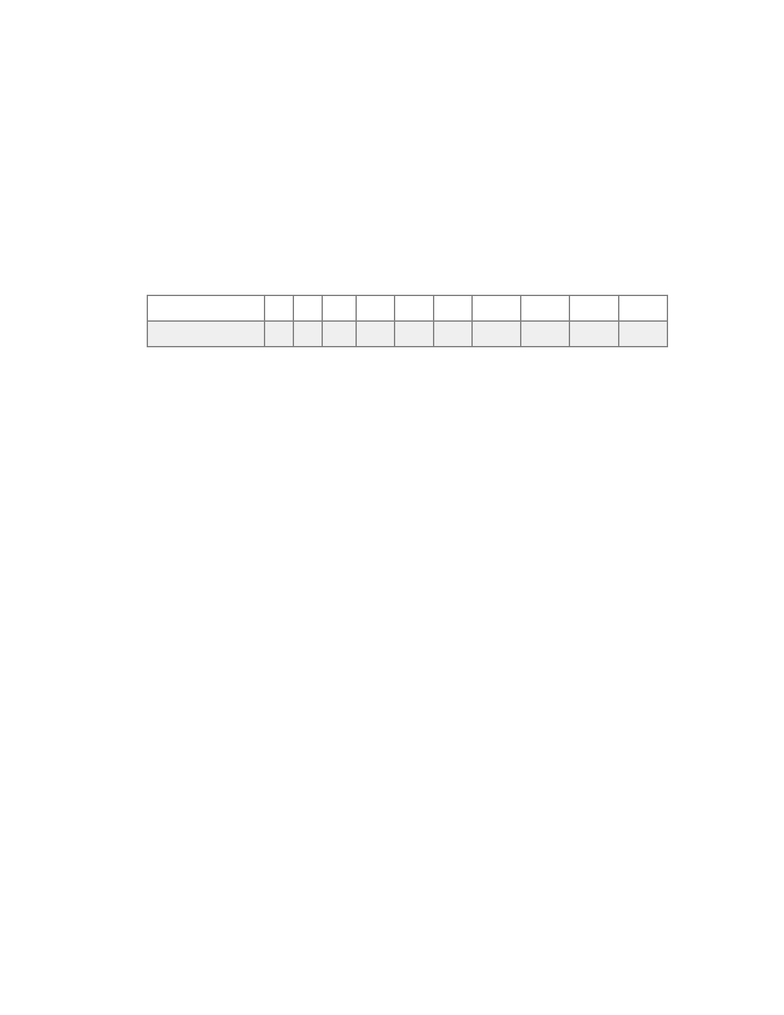# STAT 213 Chapter Notes - Chapter 9: Scatter Plot, Absorbance, Supermodel

35 views15 pagesChapter 8: Simple Linear Regression
1. Explain what each correlation coefficient means.
a. r = 0.1
b. r = - 0.15
c. r = - 1.0
d. r = 0.92
2. Match the correlation coefficients with their scatterplots. Select
the letter of the scatterplot below which corresponds to the
correlation coefficient.
a. r = -0.74
b. r = 0.22
c. r = 0.89
d. r = -0.49
Unlock document

This preview shows pages 1-3 of the document.
Unlock all 15 pages and 3 million more documents.Chapter 8: Simple Linear Regression
3. Keeping water supplies clean requires regular measurement of
levels of pollutants. The measurements are indirect- a typical
analysis involves forming a dye by a chemical reaction with the
dissolved pollutant, then passing light through the solution and
measuring its " absorbance." To calibrate such measurements, the
laboratory measures known standard solutions and uses regression
to relate absorbance and pollutant concentration. This is usually
done every day. Here is one series of data on the absorbance for
different levels of nitrates. Nitrates are measured in milligrams
per liter of water.
Chemical theory says that these data should lie on a straight line. If
the correlation is not at least 0.997, something went wrong and the
calibration procedure is repeated.
a. Find the correlation!r
b. Must the calibration be done again? (Answer YES or NO).!
Nitrates
75
50
125
300
300
300
800
1000
1800
2900
Absorbance
6.6
7.5
11
28.8
47.8
95.1
141.5
199.4
219.8
239.2
Unlock document

This preview shows pages 1-3 of the document.
Unlock all 15 pages and 3 million more documents.Chapter 8: Simple Linear Regression
4. What are all the values that a correlation!r!can possibly take?!
5. You have data for many years on the average price of a barrel of
oil and the average retail price of a gallon of unleaded regular
gasoline. When you make a scatterplot, the explanatory variable on
the!x!-axis:!
6. In a scatterplot of the average price of a barrel of oil and the
average retail price of a gallon of gasoline, you expect to see:
7. A researcher wishes to determine whether the rate of water flow
(in liters per second) over an experimental soil bed can be used
to predict the amount of soil washed away (in kilograms). In this
study, the explanatory variable is the:
8. A researcher measures the correlation between two variables. This
correlation tells us!
9. The Columbus Zoo conducts a study to determine whether a
household's income can be used to predict the amount of money the
household will give to the zoo's annual fund drive. The response
variable in this study is!
Unlock document

This preview shows pages 1-3 of the document.
Unlock all 15 pages and 3 million more documents.